# 图的表示形式：邻接矩阵和邻接表

• 时间:
• 浏览: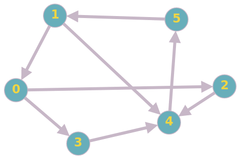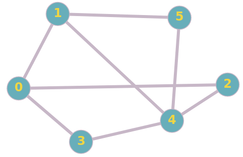1.邻接矩阵
2.邻接表

### 邻接矩阵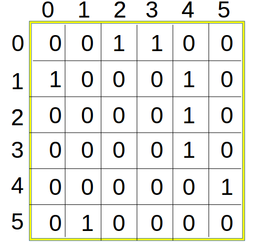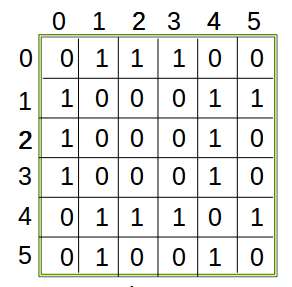````1. Create a matrix A of size NxN and initialise it with zero.`
`2. Iterate over each given edge of the form (u,v) and assign 1 to A[u][v]. Also, If graph is undirected then assign 1 to A[v][u]. `
```

``````
/*
This code is for constructing adjacency matrix for undirected graph, with minor change it will also work for directed graph.
*/
#include <bits/stdc++.h>
using namespace std;
int main()
{
// where n is number of vertices and m is number of edges
int n, u, v, m;
cin>> n>> m;
int A[n][n];
for(int i=0; i&lt;n; i++)
{
for(int j=0; i&lt;n; j++)
A[i][j]=0;
}
for(int i=0; i&lt; m; i++)
{
cin>> u>> v;
A[u][v]=1;
A[v][u]=1; // In case of directed graph this statement is removed.
}
for(int i=0; i&lt;n; i++)
{
for(int j=0; j&lt;n; j++)
cout&lt;&lt;A[i][j]&lt;&lt;" ";
cout &lt;&lt; "\n";
}
return 0;
}

``````

C ++

### 邻接表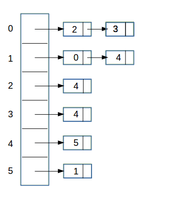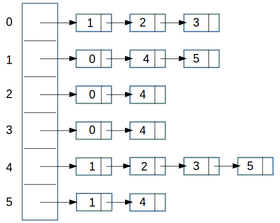````1. Create an array A of size N and type of array must be list of vertices. Intially each list is empty so each array element is initialise with empty list.`
`2. Iterate each given edge of the form (u,v) and append v to the uth list of array A. Also, If graph is undirected append u to the vth list of array A.`
```

### 执行

``````
/*
This code is for constructing adjacency list for undirected graph, with minor change it will also work for directed graph.
*/
#include <bits/stdc++.h>
using namespace std;
int main()
{
// where n is number of vertices and m is number of edges
int n, u, v, m;
cin>> n>> m;
vector< vector<int> > A(n);
for(int i=0; i< m; i++)
{
cin>> u>> v;
A[u].push_back(v);
A[v].push_back(u); // In case of directed graph this statement is removed.
}
for(int i=0; i<n; i++)
{
cout<< i<< " ----> ";
for(int j=0; j<A[i].size(); j++)
cout<< A[i][j]<< " -->";
cout <<  "\n";
}
return 0;
}
``````

### 复杂

N表示节点/顶点的数量，M表示边的数量

degree（V）表示从节点V开始的边数

### 应用

1. 邻接矩阵：邻接矩阵用于以下位置，有关每个可能的边的信息对于算法的正确工作是必需的：-Floyd-Warshall算法，其中计算从每个顶点到每个其他顶点的最短路径（如果存在）。它也可以用在DFS（深度优先搜索）和BFS（宽度优先搜索）中，但是列表在那里更有效。有时它也用于网络流中。

2. 邻接列表：邻接列表是一种节省空间的图形表示方法，如果算法不需要显式要求，则可以在几乎所有位置替换邻接矩阵。它用于诸如BFS，DFS，Dijkstra算法等的地方。